#### Question is based on the following paragraph.Wave property of electrons implies that they will show diffraction effects. Davisson and Germer demonstrated this by diffracting electrons from crystals. The law governing the diffraction from a crystal is obtained by requiring that electron waves reflected from the planes of atoms in a crystal interfere constructively (see figure).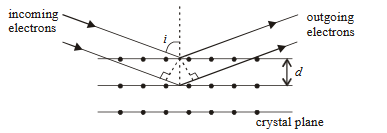Question : In an experiment, electrons are made to pass through a narrow slit of widthcomparable to their de Broglie wavelength. They are detected on a screen at a distancefrom the slit.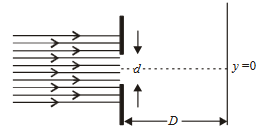Which of the following graphs can be expected to represent the number of electronsdetected as a function of the detector position(= 0 corresponds to the middle of the slit)? Option 1)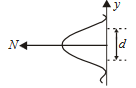Option 2)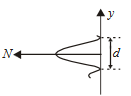Option 3)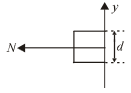Option 4)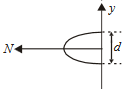As we learnt in

Davisson Germer Experiment -

A beam of electrons emitted by the electron gun is made to fall on the nickel crystal cut along cubicle at a particular angle.

- wherein

The scattered electron is received by the detector which can be rotated at any angle.

Davisson Germer Experiment -

The energy of the incident beam of electrons can be varied by changing the applied voltage to the electron gun.

- wherein

Intensity of scattered beam of electrons is found to be maximum when angle of scattering isand the accelerating potential is 54 V

As number of electron decreases both side of central maximum symmetrically.

Correct graph is 1.

Option 1)This is the correct option.

Option 2)This is an incorrect option.

Option 3)This is an incorrect option.

Option 4)This is an incorrect option.

#### perimeter